Question

# The long-run aggregate supply curve shows that by itself a permanent change in aggregate demand would lead to a long-run...

The long-run aggregate supply curve shows that by itself a permanent change in aggregate demand would lead to a long-run change

 a. in the price level and output.
 b. in the price level, but not output.
 c. in output, but not the price level.
 d. in neither the price level nor output

Option b

in the price level, but not output

the long-run aggregate supply curve shows the potential output which is produced using all the available resources at optimum level and it changes if there is a change in the available resources or technology or both but it does not change because of change in aggregate demand as the curve is vertical so if AD shifts right which only increases price and potential output will be same.

#### Earn Coins

Coins can be redeemed for fabulous gifts.

Similar Homework Help Questions
• ### 31. Which one of the following would not shift the aggregate demand curve? a. a change...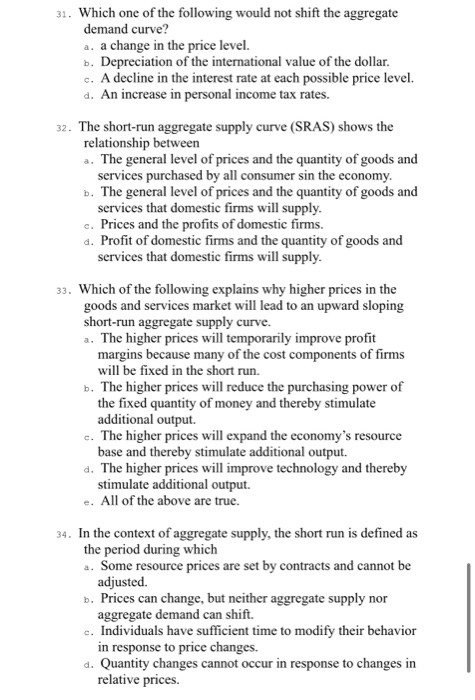31. Which one of the following would not shift the aggregate demand curve? a. a change in the price level. b. Depreciation of the international value of the dollar c. A decline in the interest rate at each possible price level. d. An increase in personal income tax rates. 32. The short-run aggregate supply curve (SRAS) shows the relationship between The general level of prices and the quantity of goods and services purchased by all consumer sin the economy. b....

• ### The​ short-run aggregate supply curve shows the​ short-run relationship between the A. price level and quantity...

The​ short-run aggregate supply curve shows the​ short-run relationship between the A. price level and quantity supplied in one market. B. price level and total demand in the entire economy. C. price level and the willingness of firms to supply output to the economy. D. consumption level and the price level. Evidence about the behavior of prices in the economy suggests that changes in aggregate demand have a relatively （Large or small） effect on prices within a few quarters so...

• ### The following graph shows the short-run aggregate supply curve (AS), the aggregate demand curve (AD), and the long-run aggregate supply curve (LRAS) for a hypothetical economy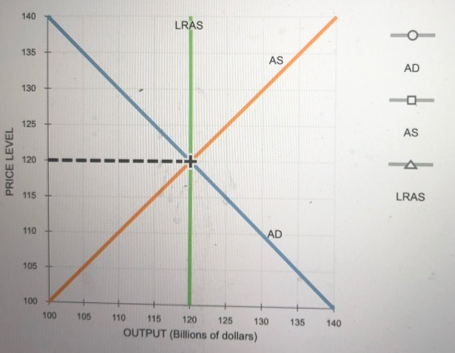9. Economic fluctuations II The following graph shows the short-run aggregate supply curve (AS), the aggregate demand curve (AD), and the long-run aggregate supply curve (LRAS) for a hypothetical economy. Initially, the expected price level is equal to the actual price level, and the economy is in long-run equilibrium at its natural level of output, \$120 billion. Suppose a bout of severe weather drives up agricultural costs, increases the costs of transporting goods and services, and increases the costs of producing goods...

• ### Describe the​ short-run aggregate supply ​(SRAS​) curve and the​ long-run aggregate supply ​(LRAS​) curve. A. the...

Describe the​ short-run aggregate supply ​(SRAS​) curve and the​ long-run aggregate supply ​(LRAS​) curve. A. the SRAS curve is horizontal and the LRAS curve is upward sloping B. the SRAS curve is horizontal and the LRAS curve is vertical C. the SRAS curve is vertical and the LRAS curve is horizontal D. the SRAS curve is vertical and the LRAS curve is upward sloping Why is the​ short-run aggregate supply curve​ horizontal? A. because output is fixed in the short...

• ### The long-run aggregate supply curve is vertical because Select one: a. potential GDP is independent of...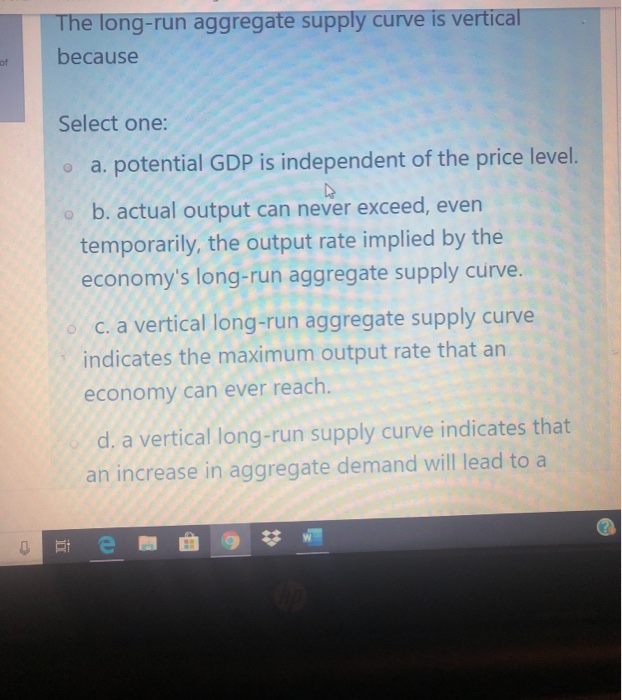The long-run aggregate supply curve is vertical because Select one: a. potential GDP is independent of the price level. ob. actual output can never exceed, even temporarily, the output rate implied by the economy's long-run aggregate supply curve. c. a vertical long-run aggregate supply curve indicates the maximum output rate that an economy can ever reach. d. a vertical long-run supply curve indicates that an increase in aggregate demand will lead to a

• ### When the aggregate demand curve and the short-run aggregate supply curve intersect, a) the long-run aggregate...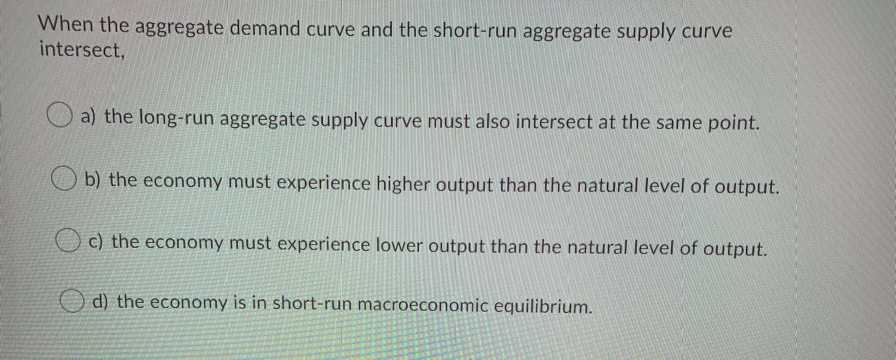When the aggregate demand curve and the short-run aggregate supply curve intersect, a) the long-run aggregate supply curve must also intersect at the same point. Ob) the economy must experience higher output than the natural level of output. o c) the economy must experience lower output than the natural level of output. o d) the economy is in short-run macroeconomic equilibrium. In a small economy in 2016, aggregate expenditure was \$900 million while GDP that year was \$750 million. Which...

• ### The economy's long-run equilibrium is at the point where the aggregate demand curve intersects the long-run...

The economy's long-run equilibrium is at the point where the aggregate demand curve intersects the long-run aggregate supply curve. True False The aggregate supply curve shows the relationship between real GDP and the average price level. True False

• ### 1. In addition to the price level, what does the aggregate demand and aggregate supply model...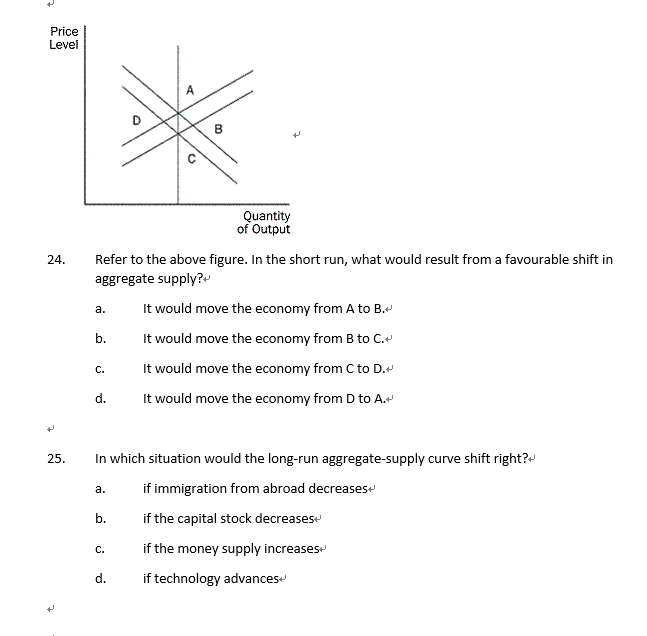1. In addition to the price level, what does the aggregate demand and aggregate supply model focus on?         a.    real GDP         b.    nominal GDP         c.     the real interest rate         d.    stock prices 2. Which statement best characterizes the long-run aggregate-supply curve?         a.    It is horizontal.         b.    It shows a positive relationship between price level and output.         c.     It demonstrates the importance of money in the economy....

• ### 1. Aggregate supply definitions The short-run aggregate supply curve shows: What happens to output in an...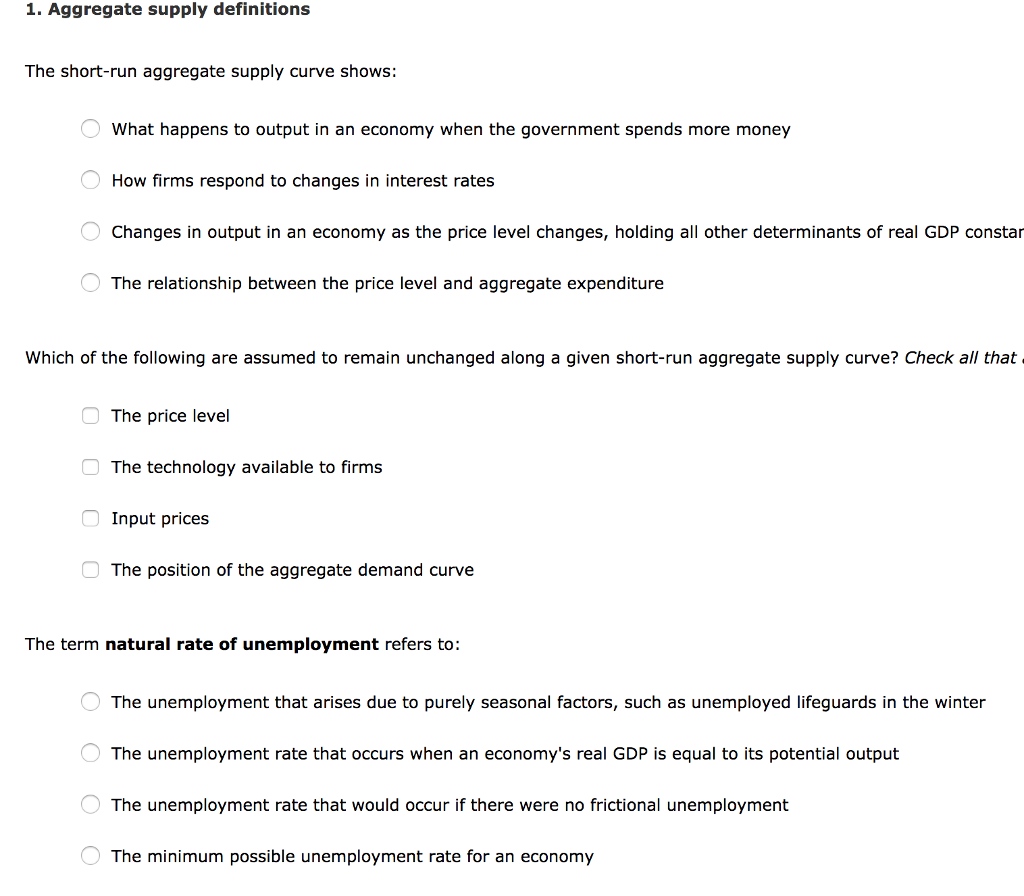1. Aggregate supply definitions The short-run aggregate supply curve shows: What happens to output in an economy when the government spends more money How firms respond to changes in interest rates Changes in output in an economy as the price level changes, holding all other determinants of real GDP constar The relationship between the price level and aggregate expenditure Which of the following are assumed to remain unchanged along a given short-run aggregate supply curve? Check all that The price...

• ### QUESTION 25 Which of the following best describes the long run in terms of aggregate supply?...

QUESTION 25 Which of the following best describes the long run in terms of aggregate supply? a. The long-run aggregate supply curve is horizontal. b. The long-run aggregate supply slopes upwards, but is NOT vertical. c. The long-run aggregate supply slopes downwards. d. The long-run aggregate supply curve is vertical. Question 39 Which of the following best characterizes market equilibrium? a. when there is no incentive for consumers or producers to change their current behaviour b. when producers earn profits...